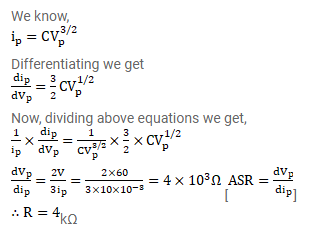# A plate current of 10mA is obtained when 60volts are applied across a diode tube.

Question:

A plate current of $10 \mathrm{~mA}$ is obtained when 60 volts are applied across a diode tube. Assuming the Langmuir-Child equation $i_{p} \propto V_{p}^{3 / 2}$

to hold, find the dynamic resistance $r_{p}$ in this operating condition.

Solution: